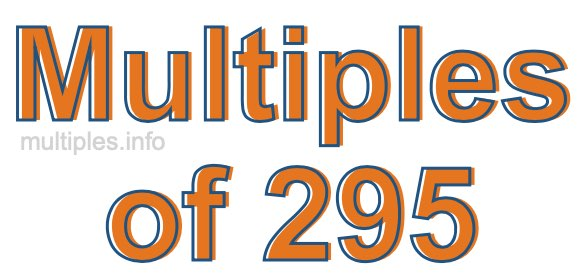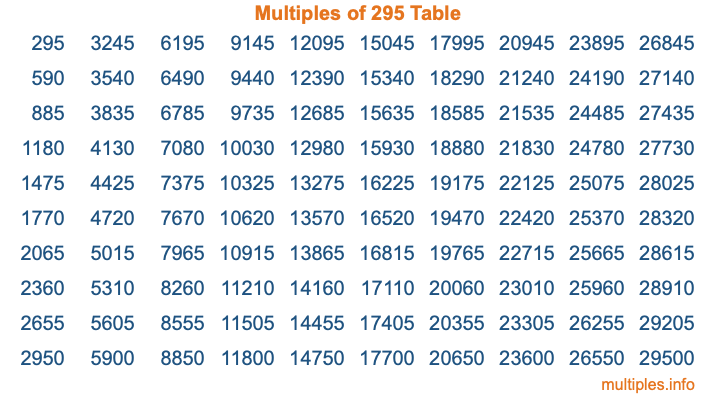Multiples of 295Welcome to the Multiples of 295 page. Here we will first teach you everything you will ever need to know about the multiples of 295, and then give you a study guide summary of everything we taught you to make sure you remember it all. Use this page to look up facts and learn information about the multiples of 295. This page will make you a multiples of two hundred ninety-five expert!

Definition of Multiples of 295
Multiples of 295 are all the numbers that when divided by 295 equal an integer. Each of the multiples of 295 are called a multiple. A multiple of 295 is created by multiplying 295 by an integer.

Therefore, to create a list of multiples of 295, you start with 1 multiplied by 295, then 2 multiplied by 295, then 3 multiplied by 295, and so on for as long as you want. Thus, the list of the first five multiples of 295 is 295, 590, 885, 1180, and 1475. To see a larger list of multiples of 295, see the printable image of Multiples of 295 further down on this page. We also have a category where you can choose any nth multiple of 295.

Multiples of 295 Checker
The Multiples of 295 Checker below checks to see if any number of your choice is a multiple of 295. In other words, it checks to see if there is any number (integer) that when multiplied by 295 will equal your number. To do that, we divide your number by 295. If the the quotient is an integer, then your number is a multiple of 295.

Is  a multiple of 295?

Least Common Multiple of 295 and ...
A Least Common Multiple (LCM) is the lowest multiple that two or more numbers have in common. This is also called the smallest common multiple or lowest common multiple and is useful to know when you are adding our subtracting fractions. Enter one or more numbers below (295 is already entered) to find the LCM.

Check out our LCM Calculator if you need more details about the Least Common Multiple or if you need the LCM for different numbers for adding and subtraction fractions.

nth Multiple of 295
As we stated above, 295 is the first multiple of 295, 590 is the second multiple of 295, 885 is the third multiple of 295, and so on. Enter a number below to find the nth multiple of 295.

th multiple of 295

Multiples of 295 vs Factors of 295
295 is a multiple of 295 and a factor of 295, but that is where the similarities end. All postive multiples of 295 are 295 or greater than 295. All positive factors of 295 are 295 or less than 295.

Below is the beginning list of multiples of 295 and the factors of 295 so you can compare:

Multiples of 295: 295, 590, 885, 1180, 1475, etc.

Factors of 295: 1, 5, 59, 295

As you can see, the multiples of 295 are all the numbers that you can divide by 295 to get a whole number. The factors of 295, on the other hand, are all the whole numbers that you can multiply by another whole number to get 295.

It's also interesting to note that if a number (x) is a factor of 295, then 295 will also be a multiple of that number (x).

Multiples of 295 vs Divisors of 295
The divisors of 295 are all the integers that 295 can be divided by evenly. Below is a list of the divisors of 295.

Divisors of 295: 1, 5, 59, 295

The interesting thing to note here is that if you take any multiple of 295 and divide it by a divisor of 295, you will see that the quotient is an integer.

Multiples of 295 Table
Below is an image of the first 100 multiples of 295 in a table. The table is in chronological order, column by column. The first column has the first ten multiples of 295, the second column has the next ten multiples of 295, and so on.The Multiples of 295 Table is also referred to as the 295 Times Table or Times Table of 295. You are welcome to print out our table for your studies.

Negative Multiples of 295
Although not often discussed or needed in math, it is worth mentioning that you can make a list of negative multiples of 295 by multiplying 295 by -1, then by -2, then by -3, and so on, to get the following list of negative multiples of 295:

-295, -590, -885, -1180, -1475, etc.

Multiples of 295 Summary
Below is a summary of important Multiples of 295 facts that we have discussed on this page. To retain the knowledge on this page, we recommend that you read through the summary and explain to yourself or a study partner why they hold true.

There are an infinite number of multiples of 295.

A multiple of 295 divided by 295 will equal a whole number.

295 divided by a factor of 295 equals a divisor of 295.

The nth multiple of 295 is n times 295.

The largest factor of 295 is equal to the first positive multiple of 295.

295 is a multiple of every factor of 295.

295 is a multiple of 295.

A multiple of 295 divided by a divisor of 295 equals an integer.

295 divided by a divisor of 295 equals a factor of 295.

Any integer times 295 will equal a multiple of 295.

Multiples of a Number
Here you can get the multiples of another number, all with the same attention to detail as we did for multiples of 295 on this page.

Multiples of
Multiples of 296
Did you find our page about multiples of two hundred ninety-five educational? Do you want more knowledge? Check out the multiples of the next number on our list!